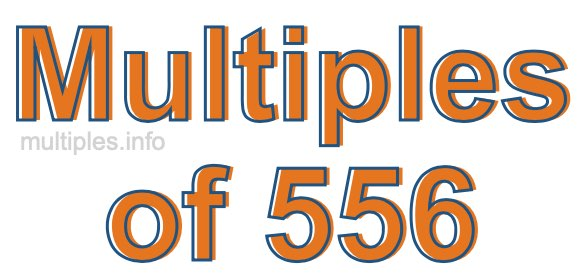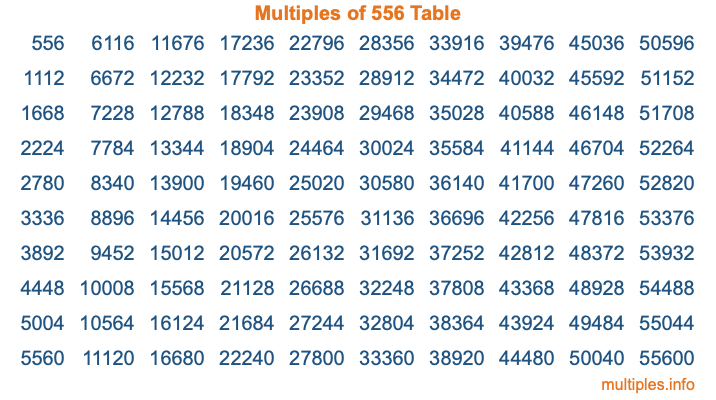Multiples of 556Welcome to the Multiples of 556 page. Here we will first teach you everything you will ever need to know about the multiples of 556, and then give you a study guide summary of everything we taught you to make sure you remember it all. Use this page to look up facts and learn information about the multiples of 556. This page will make you a multiples of five hundred fifty-six expert!

Definition of Multiples of 556
Multiples of 556 are all the numbers that when divided by 556 equal an integer. Each of the multiples of 556 are called a multiple. A multiple of 556 is created by multiplying 556 by an integer.

Therefore, to create a list of multiples of 556, you start with 1 multiplied by 556, then 2 multiplied by 556, then 3 multiplied by 556, and so on for as long as you want. Thus, the list of the first five multiples of 556 is 556, 1112, 1668, 2224, and 2780. To see a larger list of multiples of 556, see the printable image of Multiples of 556 further down on this page. We also have a category where you can choose any nth multiple of 556.

Multiples of 556 Checker
The Multiples of 556 Checker below checks to see if any number of your choice is a multiple of 556. In other words, it checks to see if there is any number (integer) that when multiplied by 556 will equal your number. To do that, we divide your number by 556. If the the quotient is an integer, then your number is a multiple of 556.

Is  a multiple of 556?

Least Common Multiple of 556 and ...
A Least Common Multiple (LCM) is the lowest multiple that two or more numbers have in common. This is also called the smallest common multiple or lowest common multiple and is useful to know when you are adding our subtracting fractions. Enter one or more numbers below (556 is already entered) to find the LCM.

Check out our LCM Calculator if you need more details about the Least Common Multiple or if you need the LCM for different numbers for adding and subtraction fractions.

nth Multiple of 556
As we stated above, 556 is the first multiple of 556, 1112 is the second multiple of 556, 1668 is the third multiple of 556, and so on. Enter a number below to find the nth multiple of 556.

th multiple of 556

Multiples of 556 vs Factors of 556
556 is a multiple of 556 and a factor of 556, but that is where the similarities end. All postive multiples of 556 are 556 or greater than 556. All positive factors of 556 are 556 or less than 556.

Below is the beginning list of multiples of 556 and the factors of 556 so you can compare:

Multiples of 556: 556, 1112, 1668, 2224, 2780, etc.

Factors of 556: 1, 2, 4, 139, 278, 556

As you can see, the multiples of 556 are all the numbers that you can divide by 556 to get a whole number. The factors of 556, on the other hand, are all the whole numbers that you can multiply by another whole number to get 556.

It's also interesting to note that if a number (x) is a factor of 556, then 556 will also be a multiple of that number (x).

Multiples of 556 vs Divisors of 556
The divisors of 556 are all the integers that 556 can be divided by evenly. Below is a list of the divisors of 556.

Divisors of 556: 1, 2, 4, 139, 278, 556

The interesting thing to note here is that if you take any multiple of 556 and divide it by a divisor of 556, you will see that the quotient is an integer.

Multiples of 556 Table
Below is an image of the first 100 multiples of 556 in a table. The table is in chronological order, column by column. The first column has the first ten multiples of 556, the second column has the next ten multiples of 556, and so on.The Multiples of 556 Table is also referred to as the 556 Times Table or Times Table of 556. You are welcome to print out our table for your studies.

Negative Multiples of 556
Although not often discussed or needed in math, it is worth mentioning that you can make a list of negative multiples of 556 by multiplying 556 by -1, then by -2, then by -3, and so on, to get the following list of negative multiples of 556:

-556, -1112, -1668, -2224, -2780, etc.

Multiples of 556 Summary
Below is a summary of important Multiples of 556 facts that we have discussed on this page. To retain the knowledge on this page, we recommend that you read through the summary and explain to yourself or a study partner why they hold true.

There are an infinite number of multiples of 556.

A multiple of 556 divided by 556 will equal a whole number.

556 divided by a factor of 556 equals a divisor of 556.

The nth multiple of 556 is n times 556.

The largest factor of 556 is equal to the first positive multiple of 556.

556 is a multiple of every factor of 556.

556 is a multiple of 556.

A multiple of 556 divided by a divisor of 556 equals an integer.

556 divided by a divisor of 556 equals a factor of 556.

Any integer times 556 will equal a multiple of 556.

Multiples of a Number
Here you can get the multiples of another number, all with the same attention to detail as we did for multiples of 556 on this page.

Multiples of
Multiples of 557
Did you find our page about multiples of five hundred fifty-six educational? Do you want more knowledge? Check out the multiples of the next number on our list!